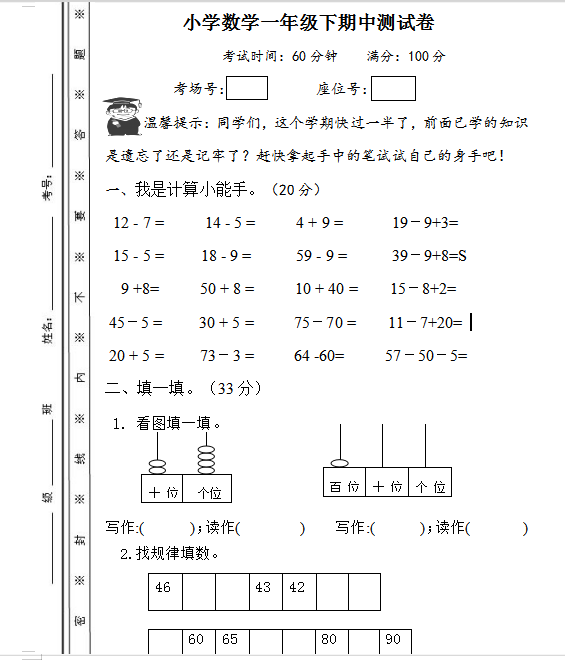• 我是计算小能手。（20分）

12 – 7 =         14 – 5 =          4 + 9 =          19－9+3=

15 – 5 =        18 – 9 =         59 – 9 =       39－9+8=S

9 +8=         50 + 8 =          10 + 40 =           15－8+2=